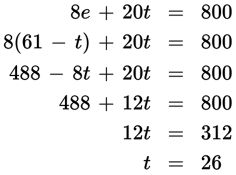# SAT Math Multiple Choice Question 400: Answer and Explanation

### Test Information

Question: 400

10. A mailing supply store sells small shipping boxes in packs of 8 or 20. If the store has 61 packs in stock totaling 800 small shipping boxes, how many packs have 20 boxes in them, assuming all the packs are full?

• A. 26
• B. 32
• C. 35
• D. 40

Explanation:

A

Difficulty: Medium

Category: Heart of Algebra / Systems of Linear Equations

Strategic Advice: Create a system of linear equations where e represents the number of packs with 8 boxes and t represents the number of packs with 20 boxes. Before selecting your final answer, make sure you answered the right question (the number of packs that have 20 boxes).

Getting to the Answer: The first equation should represent the total number of packs, e + t = 61. The second equation should represent the total number of boxes. Because e represents packs with 8 boxes and t represents packs with 20 boxes, the second equation should be 8e + 20t = 800. Now, solve the system using substitution. Solve the first equation to find that e = 61 - t. Then, substitute the result into the second equation:We assigned the variable t to the number of packs with 20 boxes, so 26 packs have 20 boxes. This is what the question asks for, so you don't need to find the value of e.